# 为什么神经网络做不了2次函数拟合，网上的都是骗人的吗？## 一、代码复现：

(204条消息) tensorflow2.0实现简单曲线拟合_一只双鱼儿的博客-CSDN博客_tensorflow2 曲线拟合

``````model = tf.keras.Sequential([
tf.keras.layers.Dense(10,input_shape=(1,),activation="elu"),
tf.keras.layers.Dense(1)
])
``````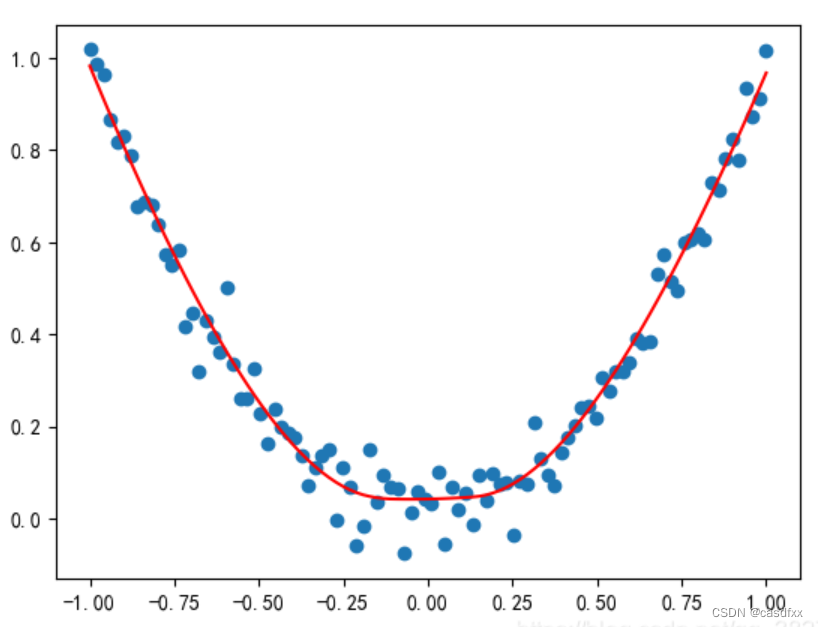可以看出，在训练集内效果是很好的，但是博主没有使用训练集外的数据。于是我扩展了预测集，效果如下：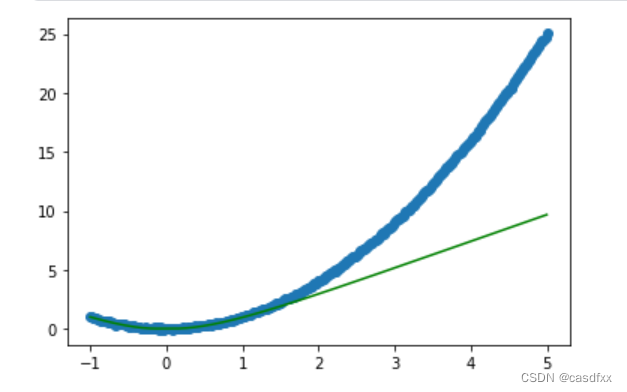``````"""
model = tf.keras.Sequential([
tf.keras.layers.Dense(10,input_shape=(1,),activation="elu"),
tf.keras.layers.Dense(1)
])
"""
model = tf.keras.Sequential([
tf.keras.layers.Dense(10,input_shape=(1,)),
tf.keras.layers.Dense(1)
])``````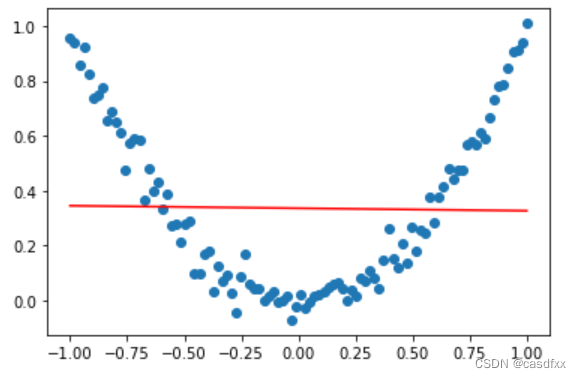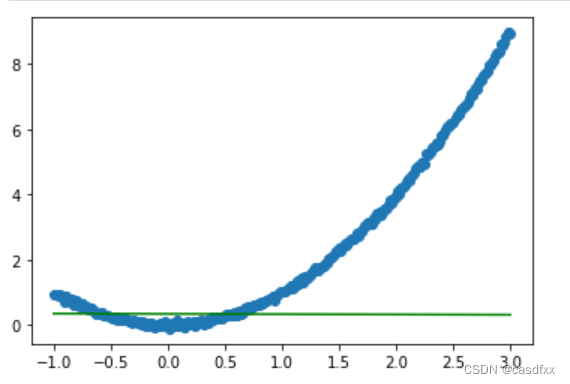好吧，那第一个问题是解决了。activation="elu"效果是很明显的。

## 二、为什么在训练集之外，生成了直线？

``````ds_x = []
ds_y = []

# 生成数据集
ds_x = np.linspace(-1,1,100)
ds_y = 5*ds_x**2 + 9*ds_x + 300 + np.random.randn(100)*0.05

class model_x2(tf.keras.Model):
def __init__(self):
super(model_x2,self).__init__()
self.layer1   = tf.keras.layers.Dense(1)
self.layer1_2 = tf.keras.layers.Dense(1)
self.layer2   = tf.keras.layers.Dense(1)

def call(self,in1):
in2  = tf.keras.layers.Multiply()((in1,in1,in1)) #in1 * in1
x    = self.layer1(in2)
x1_2 = self.layer1_2(in1)
x2   = tf.keras.layers.concatenate((x,x1_2))
out  = self.layer2(x2)
return out

model = model_x2()
model.build(input_shape=(None,1))
model.summary()

los       = tf.keras.losses.MeanSquaredError()
acc       = tf.keras.metrics.MeanSquaredError()
model.compile(optimizer=opt,loss=los,metrics=acc)

model.fit(ds_x,ds_y,epochs=500)

#以下就是画图了，将数据集里【-1，1】添加到【-1，3】，多出来的【1，3】示为预测
x = np.linspace(-1,3,20000) #np.array(range(1,100,1))
#y = x**x

y_predict = model.predict(x)

ds_x = np.linspace(-1,3,100)
ds_y = 5*ds_x**2 + 9*ds_x + 300 + np.random.randn(100)*0.05

plt.scatter(ds_x,ds_y)
plt.plot(x,y_predict,'r')
plt.show()``````

#以下就是画图了，将数据集里【-1，1】添加到【-1，3】，多出来的【1，3】示为预测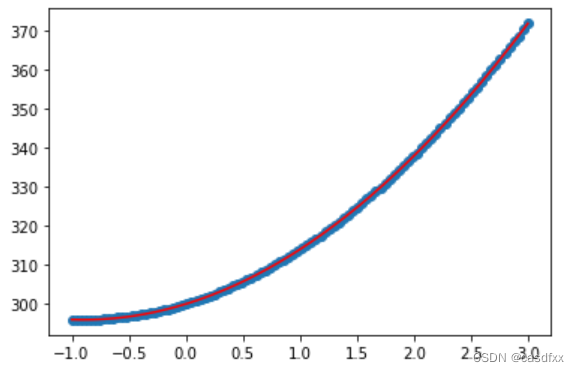NO，NO，NO

(0)